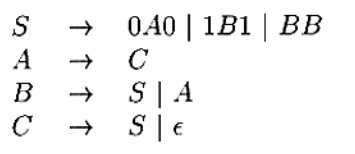# 第十章 下推自动机与CFG化简规范--习题【含答案】 软件理论基础

（1）单选题A  {S,C}

B  {S,A,B,C}

C  {S,A,C}

D  {A,B,C}

（2）单选题

A  （A,C）（C,S）

B  （A,C）（C,S）（B,S）

C  （A,C）（C,S）（B,S）（A,B）

D  {S,A,B,C}中任意一对符号都为单一偶对

（3）单选题

A  A

B  B

C  C

D  S

（4）单选题

A  终态型DPDA和空栈型DPDA的表达能力不同

B  空栈型NPDA和终态型NPDA的表达能力不同

C  空栈型DPDA和DFA的表达能力相同

D  终态型DPDA和NFA的表达能力相同

（5）单选题

A  {a*}

B  {a, ab, abc}

C  {w2w^R | w∈{0,1}*}

D  {ww^R | w∈{0,1}*}

（6）单选题

A  错误

B  正确

（7）单选题

A  L具有前缀性质，且存在DPDA P‘满足L=N(P’)

B  L不具有前缀性质，但存在DPDA P‘满足L=N(P’)

C  L不具有前缀性质，也不存在DPDA P‘满足L=N(P’)

D  L具有前缀性质，但不存在DPDA P‘满足L=N(P’)